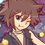# Geometry (Thailand Math POSN 2nd round)

Write a full solution.

1. Let $H$ be orthocenter of $\triangle ABC$ and point $I,J,K$ be a midpoint between each vertex $A,B,C$ and $H$ respectively. If $P$ is a point on circumcircle of $\triangle ABC$ not on the vertex $A,B,C$, and $M$ be the midpoint between $P,H$. Prove that $I,J,K,M$ lie on the same circle.

2. Let the tangents of circumcircle of $\triangle ABC$ at point $B,C$ intersect at point $D$. Prove that $\overline{AD}$ is symmedian line of $ABC$.

3. Let $P,Q$ be 2 points that are isogonal conjugate to each other in $\triangle ABC$. If $\overline{PP_{1}}$ and $\overline{QQ_{1}}$ is perpendicular to $\overline{BC}$ at point $P_{1}$ and $Q_{1}$ respectively, $\overline{PP_{2}}$ and $\overline{QQ_{2}}$ is perpendicular to $\overline{CA}$ at point $P_{2}$ and $Q_{2}$ respectively, and $\overline{PP_{3}}$ and $\overline{QQ_{3}}$ is perpendicular to $\overline{AB}$ at point $P_{3}$ and $Q_{3}$ respectively. Prove that $P_{1},P_{2},P_{3},Q_{1},Q_{2},Q_{3}$ lie on the same circle, and the center of that circle lies in the midpoint of $P$ and $Q$.

4. Let $I,N,H$ be the center of incircle, nine-point circle, and orthocenter of $\triangle ABC$ respectively. Construct $\overline{ID}, \overline{NM}$ perpendicular to $\overline{BC}$ at point $D,M$ respectively. If $\overline{AH}$ intersect circumcircle of $\triangle ABC$ at point $K$ such that $Y$ is a midpoint of $\overline{AK}$. Prove that $|ID - NM| = \displaystyle \left |r - \displaystyle \frac{AY}{2}\right|$ where $r$ is an inradius of $\triangle ABC$.

5. Let $U$ be a foot of altitude of $\triangle ABC$ from point $A$. If $U',U''$ are reflection of $U$ by $\overline{CA},\overline{AB}$ respectively, such that $\overline{U'U''}$ intersect $\overline{CA},\overline{AB}$ at point $V,W$ respectively. Prove that $\overline{BV},\overline{CW}$ is perpendicular to $\overline{CA},\overline{AB}$ respectively.

This note is a part of Thailand Math POSN 2nd round 2015.Note by Samuraiwarm Tsunayoshi
6 years, 4 months ago

This discussion board is a place to discuss our Daily Challenges and the math and science related to those challenges. Explanations are more than just a solution — they should explain the steps and thinking strategies that you used to obtain the solution. Comments should further the discussion of math and science.

When posting on Brilliant:

• Use the emojis to react to an explanation, whether you're congratulating a job well done , or just really confused .
• Ask specific questions about the challenge or the steps in somebody's explanation. Well-posed questions can add a lot to the discussion, but posting "I don't understand!" doesn't help anyone.
• Try to contribute something new to the discussion, whether it is an extension, generalization or other idea related to the challenge.

MarkdownAppears as
*italics* or _italics_ italics
**bold** or __bold__ bold
- bulleted- list
• bulleted
• list
1. numbered2. list
1. numbered
2. list
Note: you must add a full line of space before and after lists for them to show up correctly
paragraph 1paragraph 2

paragraph 1

paragraph 2

[example link](https://brilliant.org)example link
> This is a quote
This is a quote
    # I indented these lines
# 4 spaces, and now they show
# up as a code block.

print "hello world"
# I indented these lines
# 4 spaces, and now they show
# up as a code block.

print "hello world"
MathAppears as
Remember to wrap math in $$ ... $$ or $ ... $ to ensure proper formatting.
2 \times 3 $2 \times 3$
2^{34} $2^{34}$
a_{i-1} $a_{i-1}$
\frac{2}{3} $\frac{2}{3}$
\sqrt{2} $\sqrt{2}$
\sum_{i=1}^3 $\sum_{i=1}^3$
\sin \theta $\sin \theta$
\boxed{123} $\boxed{123}$

Sort by:

The first question can be solved using homothety. The points A,B,C and P lie on the circumcircle. So consider a homothetic transformation of the circumcircle about orthocenter and shrink the circle to half of its radius. The mid points of H and A,B,C, P will lie on this circle. This is the nine point circle of the triangle.

- 5 years, 7 months ago

Hi Pranav I do I know you ? I know a Pranav Rao and he is from mumbai too. This is shrihari.

- 5 years, 7 months ago